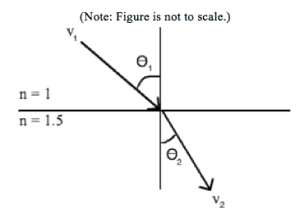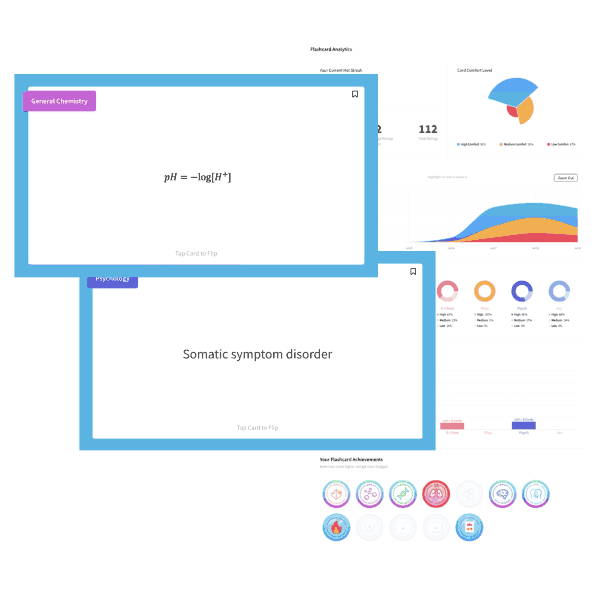# MCAT Physics Question — Snell’s Law

A beam of light is traveling through air (n = 1) when it passes through a transparent glass (n = 1.5) medium. Which of following is true concerning the angles θ and the velocity V?A. θ1 > θ2 and V1 > V2

B. θ1 > θ2 and V1 < V2

C. θ1 < θ2 and V1 > V2

D. θ1 < θ2 and V1 < V2

##### Click for Explanation

This question tests the examinees understanding of Snell’s law of refraction, n1(sinθ 1) = n2(sinθ 2). The index of refraction (n) is the ratio of the speed of light to the speed of light through a specific medium. The index of refraction for air is 1, which means V1 is equal to the speed of light (3 x 108 m/s). The refraction index for glass is 1.5, which makes

V2 = 2 x 108 m/s. Therefore, B and D are eliminated.

To compare the angle of incidence (θ 1) to the angle of refraction (θ 2), use Snell’s law to determine the ratio. Since (sinθ 1)/(sinθ 2) = n2/n1, θ1 must be greater than θ2. Thus, A is the correct answer.

## Want more MCAT practice?

### We’ve got options for every schedule and learning style!

From the best online MCAT course created by top instructors with 524+ MCAT scores to the most representative full-length practice exams and private tutoring, we can custom tailor your MCAT prep to your goals!

Not sure which option is right for you? Schedule a free MCAT consultation with an MCAT expert using the form below. No obligation, just expert advice.

Create your Free Account to access our MCAT Flashcards